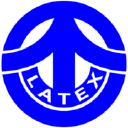# Fuji Latex Co Ltd (TSE:5199)

1 966 JPY -28 JPY ( -1.4% )Fuji Latex Co Ltd
TSE:5199

-

-

## FAQ

### What is Discount Rate?

Discount rate reflects the opportunity cost of investment (i.e. the return that could be earned on investment with similar risk).

### Cost of Equity Calculation

Cost of Equity
-
Rf
-
Beta
-
ERP
-

The Cost of Equity for Fuji Latex Co Ltd (TSE:5199) calculated via CAPM (Capital Asset Pricing Model) is -.

### WACC Calculation

WACC
-

Cost of Equity
-
Equity Weight
-

Cost of Debt
-
Debt Weight
-

The WACC for Fuji Latex Co Ltd (TSE:5199) is -.